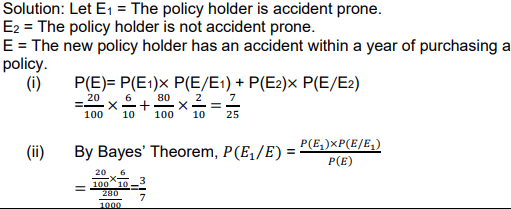# Mathematics NCERT SAMPLE QUESTION PAPER-SEMESTER-II Code(041) 2021-2022 Class XII

CBSE Class 12 Mathematics Sample Question Paper Term 2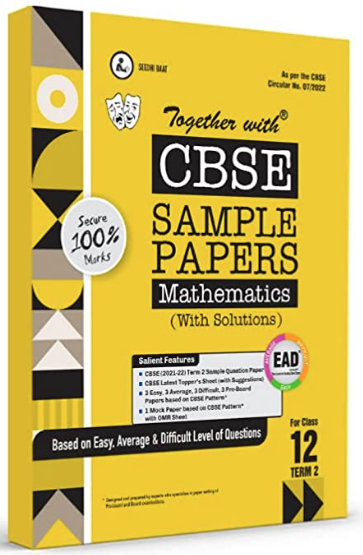## 1.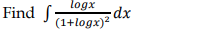OR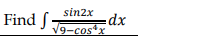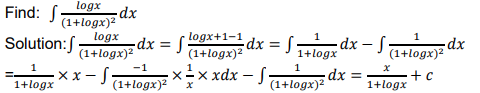OR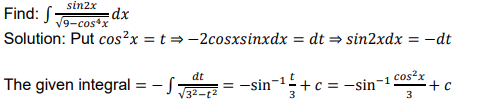## 2. Write the sum of the order and the degree of the following differential equation: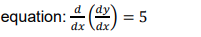### Write the sum of the order and the degree of the following differential## 3. If 𝑎̂ and 𝑏̂ are unit vectors, then prove that |𝑎̂ + 𝑏̂| = 2𝑐𝑜𝑠 0/2 , where 𝜃 is the angle between them.

### Answer. If 𝑎̂ and 𝑏̂ are unit vectors, then prove that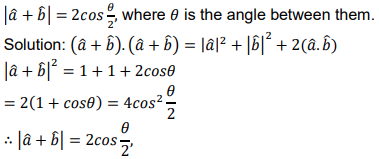## 4. Find the direction cosines of the following line: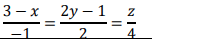### Find the direction cosines of the following line: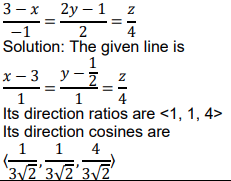## 5. A bag contains 1 red and 3 white balls. Find the probability distribution of the number of red balls if 2 balls are drawn at random from the bag one-byone without replacement.

### Answer. A bag contains 1 red and 3 white balls. Find the probability distribution of the number of red balls if 2 balls are drawn at random from the bag one-by-one without replacement. Solution: Let X be the random variable defined as the number of red balls. Then X = 0, 1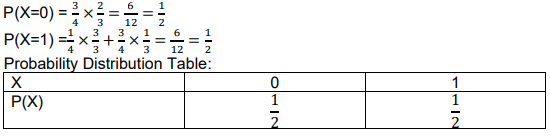## 6. Two cards are drawn at random from a pack of 52 cards one-by-one without replacement. What is the probability of getting first card red and second card Jack?

### Answer. Two cards are drawn at random from a pack of 52 cards one-by-one without replacement. What is the probability of getting first card red and second card Jack? Solution: The required probability = P((The first is a red jack card and The second is a jack card) or (The first is a red non-jack card and The second is a jack card))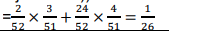## 7.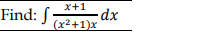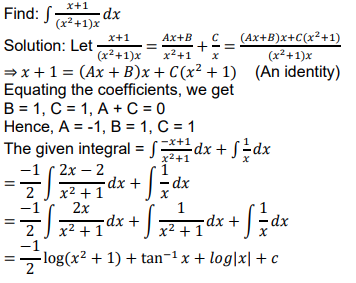## 8. Find the general solution of the following differential equation: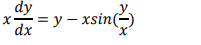OR

## Find the particular solution of the following differential equation, given that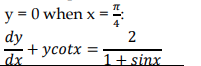###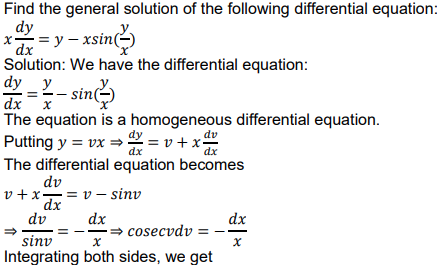𝑙𝑜𝑔|𝑐𝑜𝑠𝑒𝑐𝑣 − 𝑐𝑜𝑡𝑣| = −𝑙𝑜𝑔|𝑥| + 𝑙𝑜𝑔𝐾,𝐾 > 0 (Here, 𝑙𝑜𝑔𝐾 is an arbitrary constant.) ⇒ 𝑙𝑜𝑔|(𝑐𝑜𝑠𝑒𝑐𝑣 − 𝑐𝑜𝑡𝑣)𝑥| = 𝑙𝑜𝑔𝐾 ⇒ |(𝑐𝑜𝑠𝑒𝑐𝑣 − 𝑐𝑜𝑡𝑣)𝑥| = 𝐾 ⇒ (𝑐𝑜𝑠𝑒𝑐𝑣 − 𝑐𝑜𝑡𝑣)𝑥 = ±𝐾 ⇒ (𝑐𝑜𝑠𝑒𝑐 𝑦/𝑥− 𝑐𝑜𝑡 𝑦/𝑥) 𝑥 = 𝐶, which is the required general solution.

OR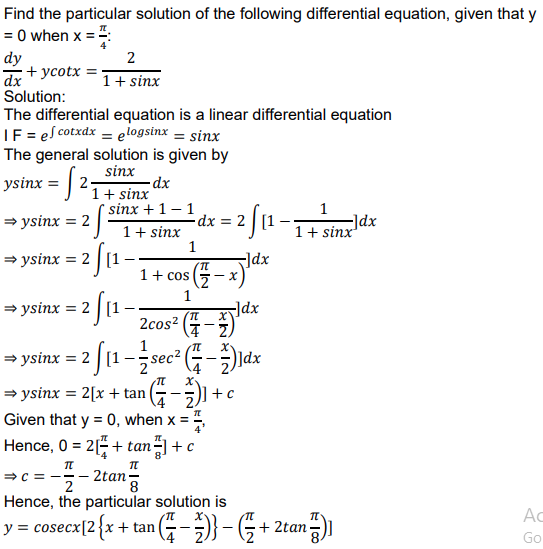OR

## Find the vector and the cartesian equations of the plane containing the point 𝑖̂+ 2𝑗̂− 𝑘̂ and parallel to the lines 𝑟⃗ = (𝑖̂+ 2𝑗̂+ 2𝑘̂) + 𝑠(2𝑖̂− 3𝑗̂+ 2𝑘̂) and 𝑟⃗ = (3𝑖̂+ 𝑗̂− 2𝑘̂) + 𝑡(𝑖̂− 3𝑗̂+ 𝑘̂)

### Answer. Find the shortest distance between the following lines: 𝑟⃗ = (𝑖̂+ 𝑗̂− 𝑘̂) + 𝑠(2𝑖̂+ 𝑗̂+ 𝑘̂) 𝑟⃗ = (𝑖̂+ 𝑗̂+ 2𝑘̂) + 𝑡(4𝑖̂+ 2𝑗̂+ 2𝑘̂)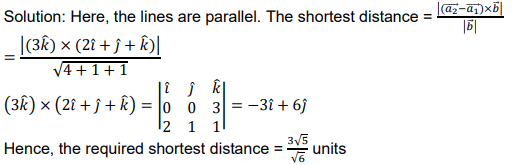OR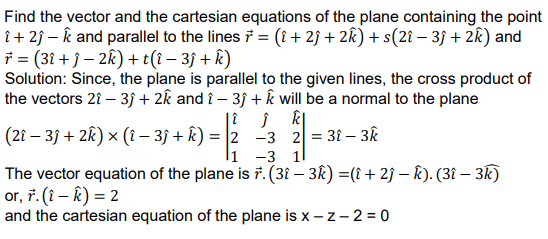## 11. Evaluate: ∫ |𝑥 3 − 3𝑥 2 + 2𝑥|𝑑�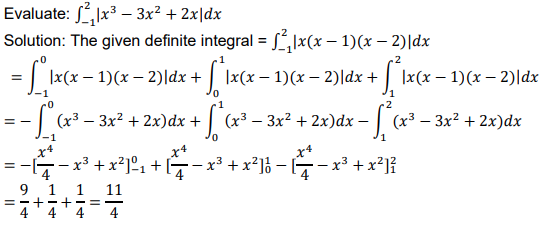OR

## Using integration, find the area of the region {(𝑥, 𝑦): 0 ≤ 𝑦 ≤ √3𝑥, 𝑥 2 + 𝑦 2 ≤ 4}

### Using integration, find the area of the region in the first quadrant enclosed by the line x + y = 2, the parabola y 2 = x and the x-axis. Solution: Solving x + y = 2 and y 2 = x simultaneously, we get the points of intersection as (1, 1) and (4, -2).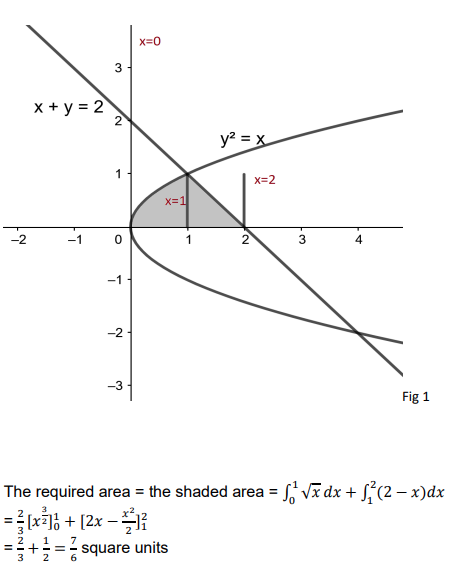OR

### Using integration, find the area of the region: {(𝑥, 𝑦): 0 ≤ 𝑦 ≤ √3𝑥, 𝑥 2 + 𝑦 2 ≤ 4}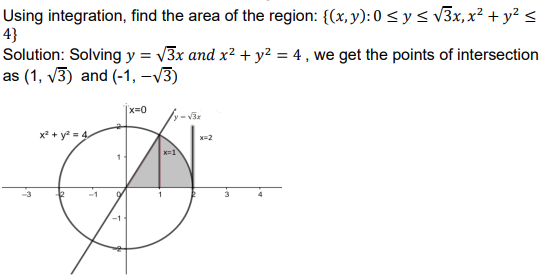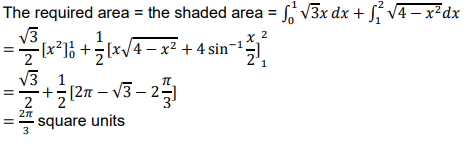## 13. Find the foot of the perpendicular from the point (1, 2, 0) upon the plane x – 3y + 2z = 9. Hence, find the distance of the point (1, 2, 0) from the given plane.

### Answer. Find the foot of the perpendicular from the point (1, 2, 0) upon the plane x – 3y + 2z = 9. Hence, find the distance of the point (1, 2, 0) from the given plane. Solution: The equation of the line perpendicular to the plane and passing through the point (1, 2, 0) is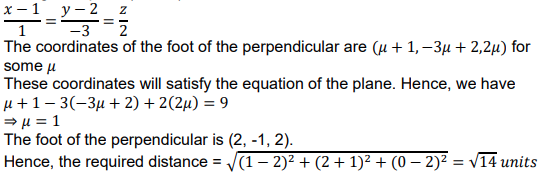## Fig 1 An insurance company believes that people can be divided into two classes: those who are accident prone and those who are not. The company’s statistics show that an accident-prone person will have an accident at sometime within a fixed one-year period with probability 0.6, whereas this probability is 0.2 for a person who is not accident prone. The company knows that 20 percent of the population is accident prone. Based on the given information, answer the followin questions. (i)what is the probability that a new policyholder will have an accident within a year of purchasing a policy? (ii) Suppose that a new policyholder has an accident within a year of purchasing a policy. What is the probability that he or she is accident prone?